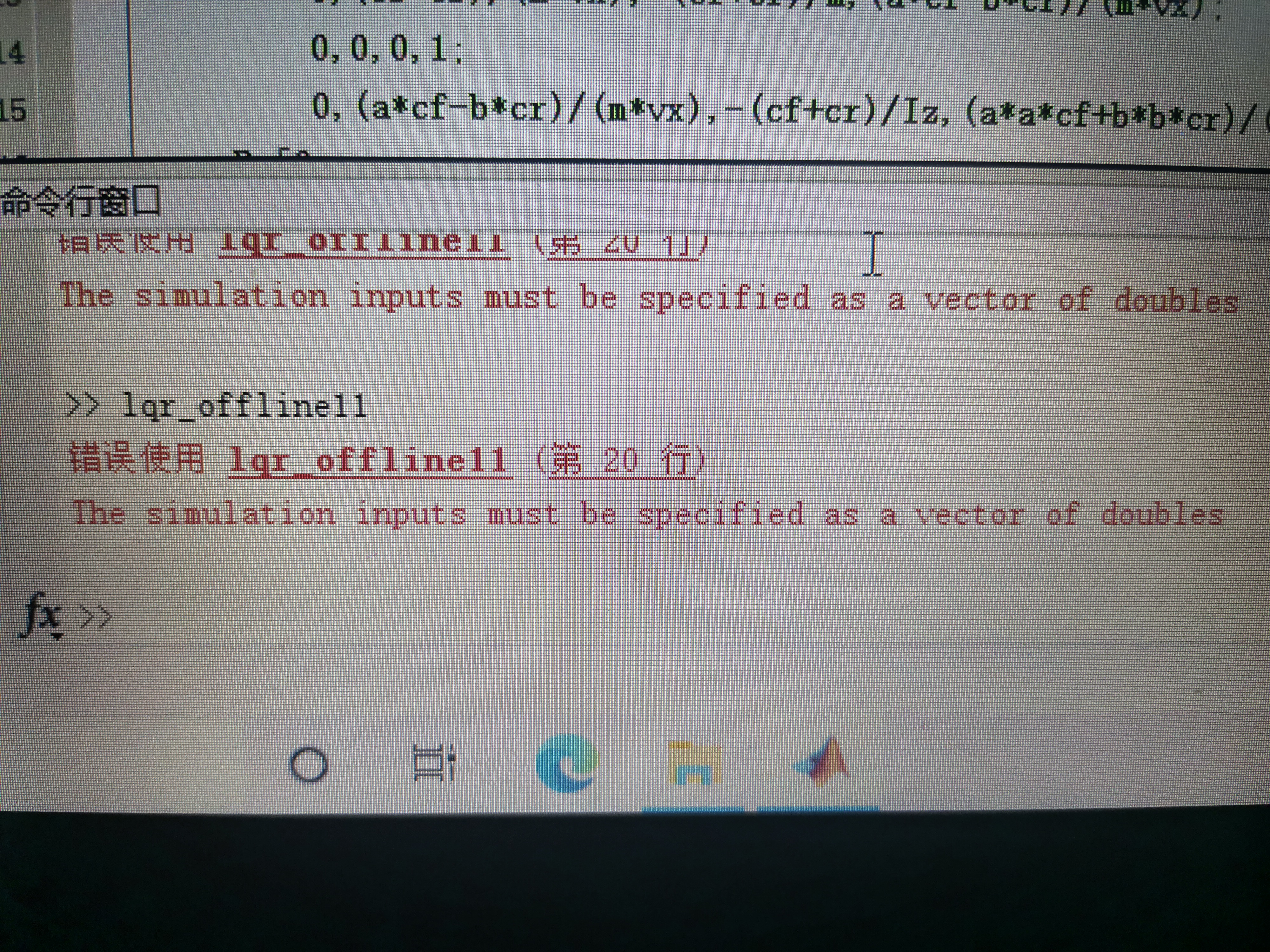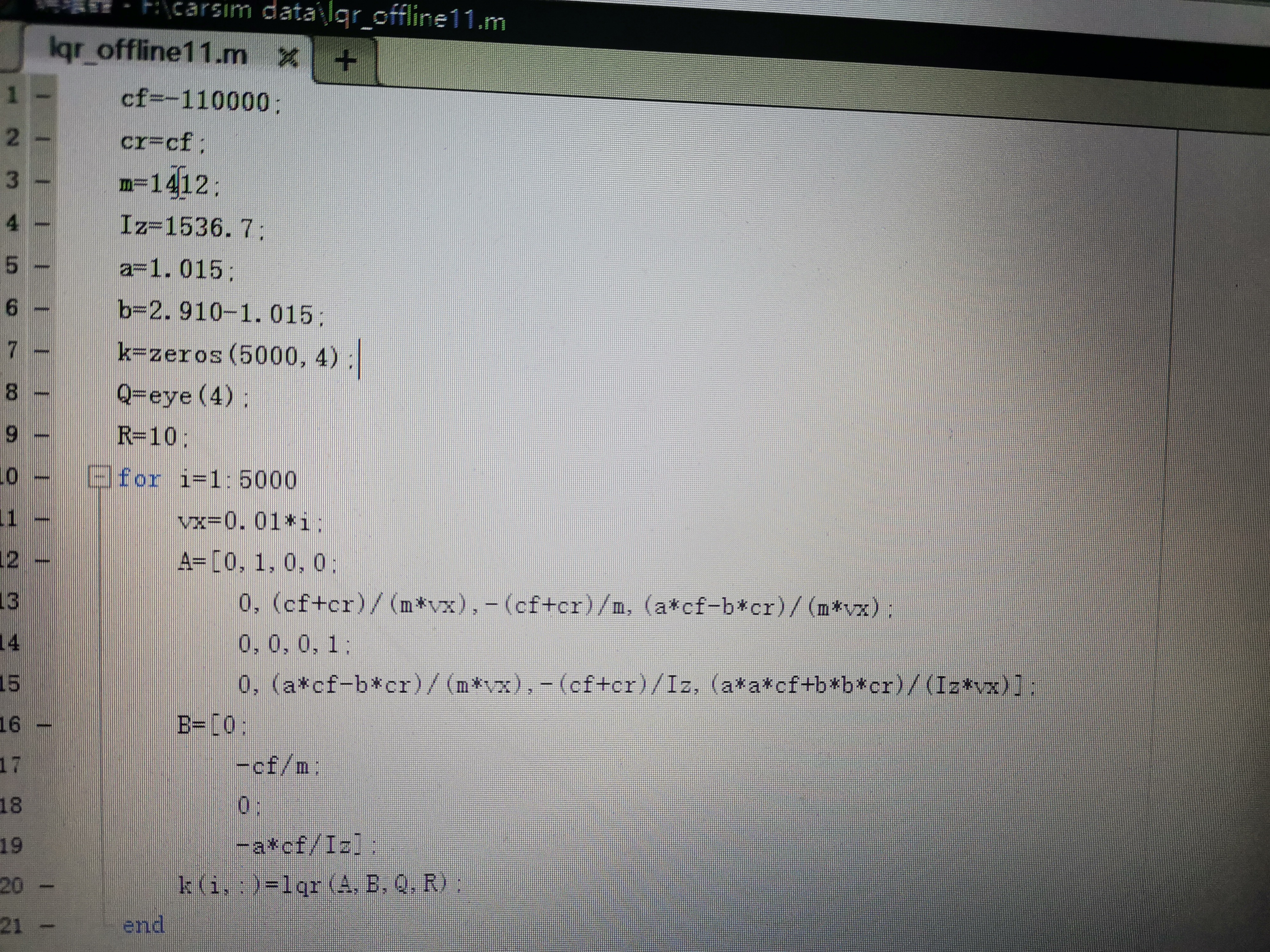2021-09-25 15:19

# lqr运行报错求解决方案

matlab运行lqr命令时会报错The simulation inputs mat be specified as a vector of doubles怎么解决，昨天换了一个matlab版本跑通了，但第二天又出现了这个错误，不知道怎么解决。• 好问题 提建议
• 收藏

#### 1条回答默认 最新

•CSDN专家-Matlab_Fans 2021-09-25 15:32
已采纳
``````
%%
cf=-110000;
%%
cr=cf;
m=1412;
Iz=1536.7;
a=1.015;
b=2.910-1.015;
k=zeros(5000,4);
Q=eye(4);
R=10;
for i=1:5000
vx=0.01*i;
A=[0,1,0,0;
0,(cf+cr)/(m*vx),-(cf+cr)/m,(a*cf-b*cr)/(m*vx);
0,0,0,1;
0,(a*cf-b*cr)/(m*vx),-(cf+cr)/Iz,(a*a*cf+b*b*cr)/(Iz*vx)];
B=[0;
-cf/m;
0;
-a*cf/Iz];
k(i,:) = lqr(A,B,Q,R);
end
``````

2020b版本运行正常，结果：

``````
k =

0.3162    0.0004    3.4888    0.0003
0.3162    0.0008    3.4888    0.0006
0.3162    0.0012    3.4888    0.0009
0.3162    0.0016    3.4888    0.0012
0.3162    0.0020    3.4888    0.0015
0.3162    0.0024    3.4888    0.0018
省略 ........
``````
已采纳该答案
评论
解决 无用
打赏 举报